# Design Optimization of a Welded Beam

This example shows how to examine the tradeoff between the strength and cost of a beam. Several publications use this example as a test problem for various multiobjective algorithms, including Deb et al.  and Ray and Liew .

For a video overview of this example, see Pareto Sets for Multiobjective Optimization.

### Problem Description

The following sketch is adapted from Ray and Liew .This sketch represents a beam welded onto a substrate. The beam supports a load P at a distance L from the substrate. The beam is welded onto the substrate with upper and lower welds, each of length l and thickness h. The beam has a rectangular cross-section, width b, and height t. The material of the beam is steel.

The two objectives are the fabrication cost of the beam and the deflection of the end of the beam under the applied load P. The load P is fixed at 6,000 lbs, and the distance L is fixed at 14 in.

The design variables are:

• x(1) = h, the thickness of the welds

• x(2) = l, the length of the welds

• x(3) = t, the height of the beam

• x(4) = b, the width of the beam

The fabrication cost of the beam is proportional to the amount of material in the beam, $\left(l+L\right)tb$, plus the amount of material in the welds, $l{h}^{2}$. Using the proportionality constants from the cited papers, the first objective is

`${F}_{1}\left(x\right)=1.10471{x}_{1}^{2}{x}_{2}+0.04811{x}_{3}{x}_{4}\left(14+{x}_{2}\right).$`

The deflection of the beam is proportional to P and inversely proportional to $b{t}^{3}$. Again, using the proportionality constants from the cited papers, the second objective is

${F}_{2}\left(x\right)=\frac{P}{{x}_{4}{x}_{3}^{3}}C$, where $C=\frac{4\left(14{\right)}^{3}}{30×1{0}^{6}}\approx 3.6587×1{0}^{-4}$ and $\mathit{P}=6,000$.

The problem has several constraints.

• The weld thickness cannot exceed the beam width. In symbols, x(1) <= x(4). In toolbox syntax:

```Aineq = [1,0,0,-1]; bineq = 0;```
• The shear stress $\tau \left(x\right)$ on the welds cannot exceed 13,600 psi. To calculate the shear stress, first calculate preliminary expressions:

`${\tau }_{1}=\frac{1}{\sqrt{2}{x}_{1}{x}_{2}}$`

`$R=\sqrt{{x}_{2}^{2}+\left({x}_{1}+{x}_{3}{\right)}^{2}}$`

`${\tau }_{2}=\frac{\left(L+{x}_{2}/2\right)R}{\sqrt{2}{x}_{1}{x}_{3}\left({x}_{2}^{2}/3+\left({x}_{1}+{x}_{3}{\right)}^{2}}$`

`$\tau \left(x\right)=P\sqrt{{\tau }_{1}^{2}+{\tau }_{2}^{2}+\frac{2{\tau }_{1}{\tau }_{2}{x}_{2}}{R}}.$`

In summary, the shear stress on the welds has the constraint $\tau \left(x\right)$ <= 13600.

• The normal stress $\sigma \left(x\right)$ on the welds cannot exceed 30,000 psi. The normal stress is $P\frac{6L}{{x}_{4}{x}_{3}^{2}}\le 30×1{0}^{3}$.

• The buckling load capacity in the vertical direction must exceed the applied load of 6,000 lbs. Using the values of Young's modulus $E=30×1{0}^{6}$ psi and $G=12×1{0}^{6}$ psi, the buckling load constraint is $\frac{4.013E{x}_{3}{x}_{4}^{3}}{6{L}^{2}}\left(1-\frac{{x}_{3}}{2L}\sqrt{\frac{E}{4G}}\right)\ge 6000$. Numerically, this becomes the inequality $64,746.022\left(1-0.0282346\phantom{\rule{0.16666666666666666em}{0ex}}{x}_{3}\right){x}_{3}{x}_{4}^{3}\ge 6000$.

• The bounds on the variables are 0.125 <=x(1) <= 5, 0.1 <= x(2) <= 10, 0.1 <= x(3) <= 10, and 0.125 <= x(4) <= 5. In toolbox syntax:

```lb = [0.125,0.1,0.1,0.125]; ub = [5,10,10,5];```

The objective functions appear at the end of this example in the function `objval(x)`. The nonlinear constraints appear at the end of this example in the function `nonlcon(x)`.

### Multiobjective Problem Formulation and `paretosearch` Solution

You can optimize this problem in several ways:

• Set a maximum deflection, and find a single-objective minimal fabrication cost over designs that satisfy the maximum deflection constraint.

• Set a maximum fabrication cost, and find a single-objective minimal deflection over designs that satisfy the fabrication cost constraint.

• Solve a multiobjective problem, visualizing the tradeoff between the two objectives.

To use the multiobjective approach, which gives more information about the problem, set the objective function and nonlinear constraint function.

```fun = @objval; nlcon = @nonlcon;```

Solve the problem using `paretosearch` with the `'psplotparetof'` plot function. To reduce the amount of diagnostic display information, set the `Display` option to `'off'`.

```opts_ps = optimoptions('paretosearch','Display','off','PlotFcn','psplotparetof'); rng default % For reproducibility [x_ps1,fval_ps1,~,psoutput1] = paretosearch(fun,4,Aineq,bineq,[],[],lb,ub,nlcon,opts_ps);````disp("Total Function Count: " + psoutput1.funccount);`
```Total Function Count: 1870 ```

For a smoother Pareto front, try using more points.

```npts = 160; % The default is 60 opts_ps.ParetoSetSize = npts; [x_ps2,fval_ps2,~,psoutput2] = paretosearch(fun,4,Aineq,bineq,[],[],lb,ub,nlcon,opts_ps);```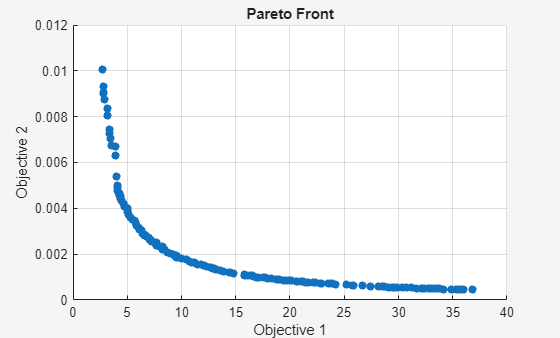`disp("Total Function Count: " + psoutput2.funccount);`
```Total Function Count: 6254 ```

This solution looks like a smoother curve, but it has a smaller extent of Objective 2. The solver takes over three times as many function evaluations when using 160 Pareto points instead of 60.

### `gamultiobj` Solution

To see if the solver makes a difference, try the `gamultiobj` solver on the problem. Set equivalent options as in the previous solution. Because the `gamultiobj` solver keeps fewer than half of its solutions on the best Pareto front, use two times as many points as before.

```opts_ga = optimoptions('gamultiobj','Display','off','PlotFcn','gaplotpareto','PopulationSize',2*npts); [x_ga1,fval_ga1,~,gaoutput1] = gamultiobj(fun,4,Aineq,bineq,[],[],lb,ub,nlcon,opts_ga);````disp("Total Function Count: " + gaoutput1.funccount);`
```Total Function Count: 38401 ```

`gamultiobj` takes tens of thousands of function evaluations, whereas `paretosearch` takes only thousands.

### Compare Solutions

The `gamultiobj` solution seems to differ from the `paretosearch` solution, although it is difficult to tell because the plotted scales differ. Plot the two solutions on the same plot, using the same scale.

```fps2 = sortrows(fval_ps2,1,'ascend'); figure hold on plot(fps2(:,1),fps2(:,2),'r-') fga = sortrows(fval_ga1,1,'ascend'); plot(fga(:,1),fga(:,2),'b--') xlim([0,40]) ylim([0,1e-2]) legend('paretosearch','gamultiobj') xlabel 'Cost' ylabel 'Deflection' hold off```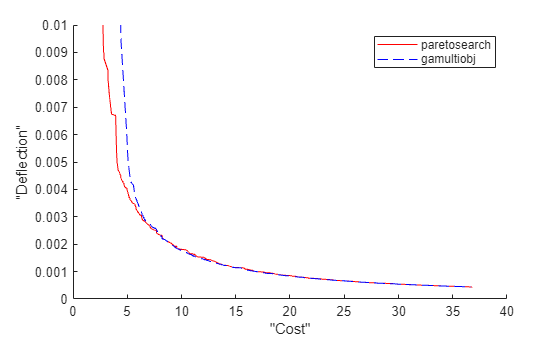The `gamultiobj` solution is better in the rightmost portion of the plot, whereas the `paretosearch` solution is better in the leftmost portion. `paretosearch` uses many fewer function evaluations to obtain its solution.

Typically, when the problem has no nonlinear constraints, `paretosearch` is at least as accurate as `gamultiobj`. However, the resulting Pareto sets can have somewhat different ranges. In this case, the presence of a nonlinear constraint causes the `paretosearch` solution to be less accurate over part of the range.

One of the main advantages of `paretosearch` is that it usually takes many fewer function evaluations.

### Start from Single-Objective Solutions

To help the solvers find better solutions, start them from points that are the solutions to minimizing the individual objective functions. The `pickindex` function returns a single objective from the `objval` function. Use `fmincon` to find single-objective optima. Then use those solutions as initial points for a multiobjective search.

```x0 = zeros(2,4); x0f = (lb + ub)/2; opts_fmc = optimoptions('fmincon','Display','off','MaxFunctionEvaluations',1e4); x0(1,:) = fmincon(@(x)pickindex(x,1),x0f,Aineq,bineq,[],[],lb,ub,@nonlcon,opts_fmc); x0(2,:) = fmincon(@(x)pickindex(x,2),x0f,Aineq,bineq,[],[],lb,ub,@nonlcon,opts_fmc);```

Examine the single-objective optima.

`objval(x0(1,:))`
```ans = 1×2 2.3810 0.0158 ```
`objval(x0(2,:))`
```ans = 1×2 76.7188 0.0004 ```

The minimum cost is 2.381, which gives a deflection of 0.158. The minimum deflection is 0.0004, which has a cost of 76.7253. The plotted curves are quite steep near the ends of their ranges, meaning you get much less deflection if you take a cost a bit above its minimum, or much less cost if you take a deflection a bit above its minimum.

Start `paretosearch` from the single-objective solutions. Because you will plot the solutions later on the same plot, remove the `paretosearch` plot function.

```opts_ps.InitialPoints = x0; opts_ps.PlotFcn = []; [x_psx0,fval_ps1x0,~,psoutput1x0] = paretosearch(fun,4,Aineq,bineq,[],[],lb,ub,nlcon,opts_ps); disp("Total Function Count: " + psoutput1x0.funccount);```
```Total Function Count: 4839 ```

Start `ga` from the same initial points, and remove its plot function.

```opts_ga.InitialPopulationMatrix = x0; opts_ga.PlotFcn = []; [~,fval_ga,~,gaoutput] = gamultiobj(fun,4,Aineq,bineq,[],[],lb,ub,nlcon,opts_ga); disp("Total Function Count: " + gaoutput.funccount);```
```Total Function Count: 37441 ```

Plot the solutions on the same axes.

```fps = sortrows(fval_ps1x0,1,'ascend'); figure hold on plot(fps(:,1),fps(:,2),'r-') fga = sortrows(fval_ga,1,'ascend'); plot(fga(:,1),fga(:,2),'b--') xlim([0,40]) ylim([0,1e-2]) legend('paretosearch','gamultiobj') xlabel 'Cost' ylabel 'Deflection' hold off```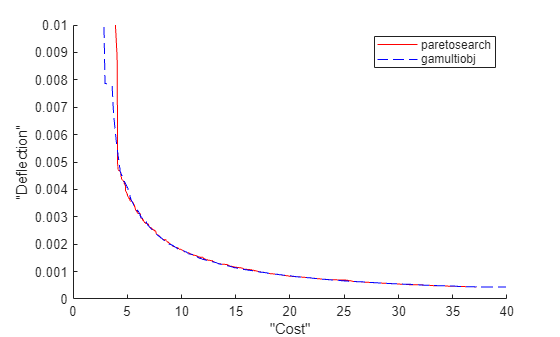By starting from the single-objective solutions, the `gamultiobj` solution is slightly better than the `paretosearch` solution throughout the plotted range. However, `gamultiobj` takes almost ten times as many function evaluations to reach its solution.

### Hybrid Function

`gamultiobj` can call the hybrid function `fgoalattain` automatically to attempt to reach a more accurate solution. See whether the hybrid function improves the solution.

```opts_ga.HybridFcn = 'fgoalattain'; [xgah,fval_gah,~,gaoutputh] = gamultiobj(fun,4,Aineq,bineq,[],[],lb,ub,nlcon,opts_ga); disp("Total Function Count: " + gaoutputh.funccount);```
```Total Function Count: 57478 ```
```fgah = sortrows(fval_gah,1,'ascend'); figure hold on plot(fps(:,1),fps(:,2),'r-') plot(fga(:,1),fga(:,2),'b--') plot(fgah(:,1),fgah(:,2),'g-') xlim([0,40]) ylim([0,1e-2]) legend('paretosearch','gamultiobj','gamultiobj/fgoalattain') xlabel 'Cost' ylabel 'Deflection' hold off```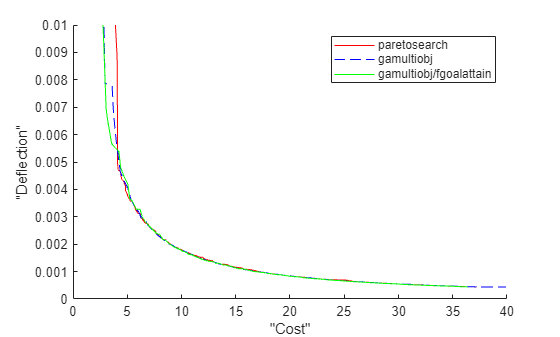The hybrid function provides a slight improvement on the `gamultiobj` solution, mainly in the leftmost part of the plot.

### Run `fgoalattain` Manually from `paretosearch` Solution Points

Although `paretosearch` has no built-in hybrid function, you can improve the `paretosearch` solution by running `fgoalattain` from the `paretosearch` solution points. Create a goal and weights for `fgoalattain` by using the same setup for `fgoalattain` as described in gamultiobj Hybrid Function.

```Fmax = max(fval_ps1x0); nobj = numel(Fmax); Fmin = min(fval_ps1x0); w = sum((Fmax - fval_ps1x0)./(1 + Fmax - Fmin),2); p = w.*((Fmax - fval_ps1x0)./(1 + Fmax - Fmin)); xnew = zeros(size(x_psx0)); nsol = size(xnew,1); fvalnew = zeros(nsol,nobj); opts_fg = optimoptions('fgoalattain','Display','off'); nfv = 0; for ii = 1:nsol [xnew(ii,:),fvalnew(ii,:),~,~,output] = fgoalattain(fun,x_psx0(ii,:),fval_ps1x0(ii,:),p(ii,:),... Aineq,bineq,[],[],lb,ub,nlcon,opts_fg); nfv = nfv + output.funcCount; end disp("fgoalattain Function Count: " + nfv)```
```fgoalattain Function Count: 14049 ```
```fnew = sortrows(fvalnew,1,'ascend'); figure hold on plot(fps(:,1),fps(:,2),'r-') plot(fga(:,1),fga(:,2),'b--') plot(fgah(:,1),fgah(:,2),'g-') plot(fnew(:,1),fnew(:,2),'k.-') xlim([0,40]) ylim([0,1e-2]) legend('paretosearch','gamultiobj','gamultiobj/fgoalattain','paretosearch/fgoalattain') xlabel 'Cost' ylabel 'Deflection'```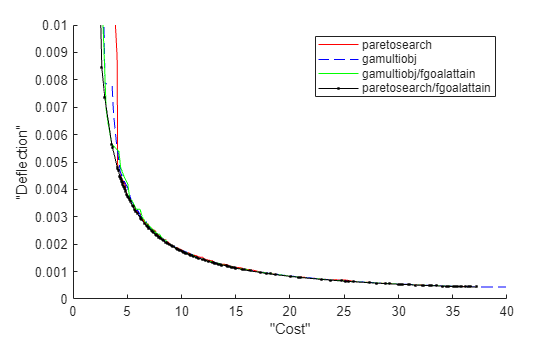The combination of `paretosearch` and `fgoalattain` creates the most accurate Pareto front. Zoom in to see.

```xlim([3.64 13.69]) ylim([0.00121 0.00442]) hold off```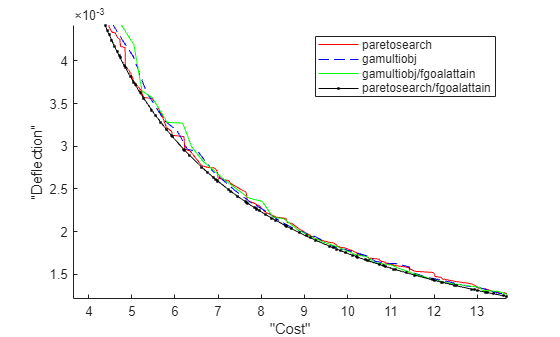Even with the extra `fgoalattain` computations, the total function count for the combination is less than half of the function count for the `gamultiobj` solution alone.

```fprintf("Total function count for gamultiobj alone is %d.\n" + ... "For paretosearch and fgoalattain together it is %d.\n",... gaoutput.funccount,nfv + psoutput1x0.funccount)```
```Total function count for gamultiobj alone is 37441. For paretosearch and fgoalattain together it is 18888. ```

### Find Good Parameters from Plot

The plotted points show the best values in function space. To determine which parameters achieve these function values, find the size of the beam and size of the weld in order to get a particular cost/deflection point. For example, the plot of `paretosearch` followed by `fgoalattain` shows points with a cost of about 6 and a deflection of about 3.5e–3. Determine the sizes of the beam and weld that achieve these points.

```whichgood = find(fvalnew(:,1) <= 6 & fvalnew(:,2) <= 3.5e-3); goodpoints = table(xnew(whichgood,:),fvalnew(whichgood,:),'VariableNames',{'Parameters' 'Objectives'})```
```goodpoints=4×2 table Parameters Objectives ________________________________________ ___________________ 0.63457 1.5187 10 0.67262 5.6974 0.0032637 0.61635 1.5708 10 0.63165 5.391 0.0034753 0.63228 1.5251 10 0.6674 5.6584 0.0032892 0.65077 1.4751 10 0.70999 5.976 0.0030919 ```

Four sets of parameters achieve a cost of less than 6 and a deflection of less than 3.5e–3:

• Weld thickness slightly over 0.6

• Weld length about 1.5

• Beam height 10 (the upper bound)

• Beam width between 0.63 and 0.71

### Objective and Nonlinear Constraints

```function [Cineq,Ceq] = nonlcon(x) sigma = 5.04e5 ./ (x(:,3).^2 .* x(:,4)); P_c = 64746.022*(1 - 0.028236*x(:,3)).*x(:,3).*x(:,4).^3; tp = 6e3./sqrt(2)./(x(:,1).*x(:,2)); tpp = 6e3./sqrt(2) .* (14+0.5*x(:,2)).*sqrt(0.25*(x(:,2).^2 + (x(:,1) + x(:,3)).^2)) ./ (x(:,1).*x(:,2).*(x(:,2).^2 / 12 + 0.25*(x(:,1) + x(:,3)).^2)); tau = sqrt(tp.^2 + tpp.^2 + (x(:,2).*tp.*tpp)./sqrt(0.25*(x(:,2).^2 + (x(:,1) + x(:,3)).^2))); Cineq = [tau - 13600,sigma - 3e4,6e3 - P_c]; Ceq = []; end function F = objval(x) f1 = 1.10471*x(:,1).^2.*x(:,2) + 0.04811*x(:,3).*x(:,4).*(14.0+x(:,2)); f2 = 2.1952./(x(:,3).^3 .* x(:,4)); F = [f1,f2]; end function z = pickindex(x,k) z = objval(x); % evaluate both objectives z = z(k); % return objective k end```

### References

 Deb, Kalyanmoy, J. Sundar, Udaya Bhaskara Rao N, and Shamik Chaudhuri. Reference Point Based Multi-Objective Optimization Using Evolutionary Algorithms. International Journal of Computational Intelligence Research, Vol. 2, No. 3, 2006, pp. 273–286. Available at https://www.softcomputing.net/ijcir/vol2-issu3-paper4.pdf

 Ray, T., and K. M. Liew. A Swarm Metaphor for Multiobjective Design Optimization. Engineering Optimization 34, 2002, pp.141–153.